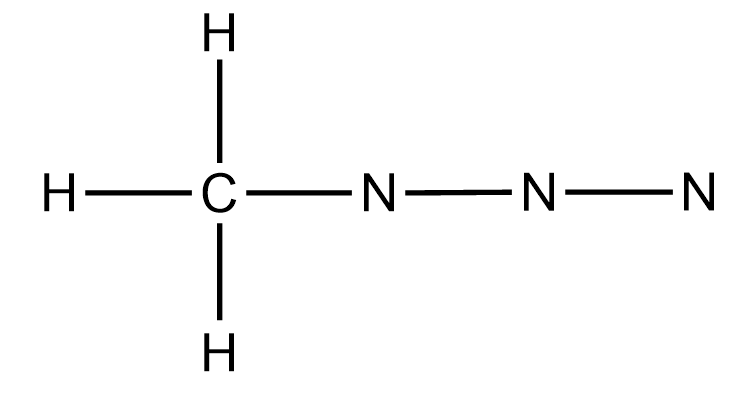# Problem: Use VSEPR to predict the geometry (including bond angles) about each interior atom of methyl azide (CH3N3), and make a sketch of the molecule. Would you expect the bond angle between the two interior nitrogen atoms to be the same or different? Would you expect the two nitrogen-nitrogen bond lengths to be the same or different?Draw the Lewis structures for the resonance forms of methyl azide (CH3N3).

###### FREE Expert Solution
• According to the provided molecular formula, the skeletal or initial structure of CH3Nwill appear as:• Calculating for the valence electrons, C appears to have 4 v.e, H as 1 and N as 5. Total valence electrons for this structure is 22. 4(1) + 1(3) + 5(3) = 22

96% (322 ratings)###### Problem Details

Use VSEPR to predict the geometry (including bond angles) about each interior atom of methyl azide (CH3N3), and make a sketch of the molecule. Would you expect the bond angle between the two interior nitrogen atoms to be the same or different? Would you expect the two nitrogen-nitrogen bond lengths to be the same or different?

Draw the Lewis structures for the resonance forms of methyl azide (CH3N3).